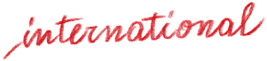# The case of maths rules

Product: Book

Trim size in cm: 21x29,7

Pages: 182

ISBN: 978-88-590-0974-0

Publication date: 01/03/2016

Suitable for: Primary 2nd level (ages 8-10)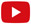Video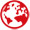Rights sold to: Slovenia

The case of maths rules for primary school has arrived! You’ll find everything you need in it for revising the rules of maths and geometry, calculation procedures and summary tables with formulas!

For example, if you don’t remember how to classify, count, compare and order natural numbers or you want to revise how powers are calculated, go to the numbers section. Or let’s say you want to know how to calculate the perimeter or area of a polygon? Look up the rule in the space and shapes section, there are pictures to help you understand better too!

It’s easy to use: in each section there are plenty of rules to revise, from the easiest to the most complex. Each rule comes with an example and an easy-to-understand definition. If you don’t remember how to do a sum, check the procedures pages: you’ll find the calculation with a step-by-step explanation!

Numbers

Natural numbers
Powers
Relative numbers
Rounding off
Multiples and divisors
Prime numbers

Operations

Subtraction
Multiplication
Division
Arithmetic expressions

Fractions

Types of fraction
Operating with fractions
Calculating percentage

Decimal numbers

Subtraction
Multiplication
Division

Measurements

Length
Capacity
Mass
Time
Value

Space and shapes

Lines, segments and angles
Polygons
Perimeter
Surface area
Regular polygons
Circle and circumference
Solids

Problems

Solving problems
Problems with fractions
Problem with percentages# Everything you need in mathematics... all in one case!

The case of maths rules

The case of maths rules contains everything you need for learning or revising the rules and definitions of maths and geometry, calculation properties and procedures, and summary tables with formulas.

#### A single book which contains all the rules and procedures for calculations.

Each page is dedicated to the explanation of a single rule or procedure and is introduced with a cartoon which illustrates the content, providing pupils with «visual hooks» which enable them to understand mathematical code better.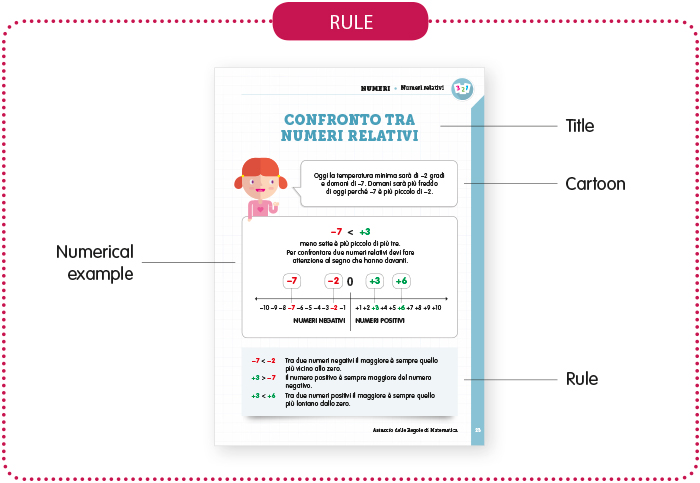Each rule is presented on the page in the same way: the title of the topic, a cartoon which introduces the application of the rule, a numerical example and the statement of the rule.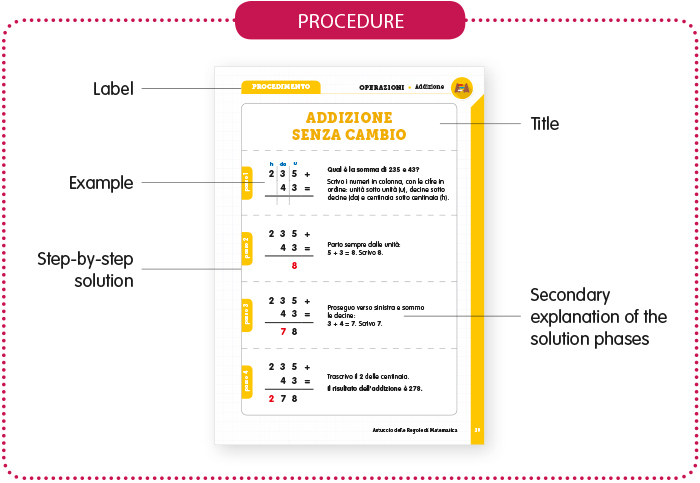The solution procedures for the calculations and problems are given step-by-step on the pages identified with the procedure label. The title on the top of the page identifies the topic, whilst the numerical example always appears alongside an explanation of what to do in each solution phase.

#### Seven topic sections for learning and revising maths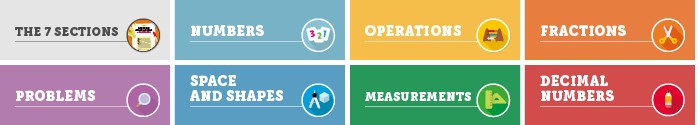Each section has a different colour and presents the main rules of the topic it deals with
All topics follow an increasing level of difficulty in the same way as they are dealt with from year one to five at school The case of maths rules is recommended for pupils with dyscalculia too. It can in fact be considered amongst "non-specific" or "functional" compensatory tools, which support deficient aspects of "transversal" skills such as memory, attention etc.

Thematic Areas